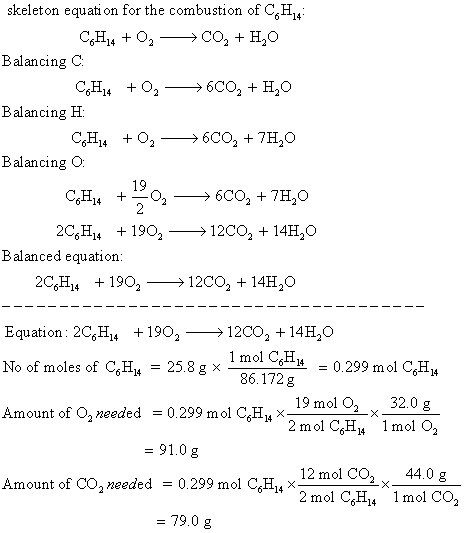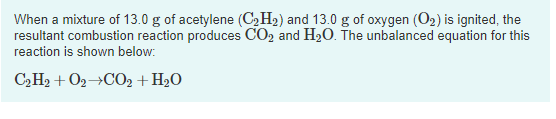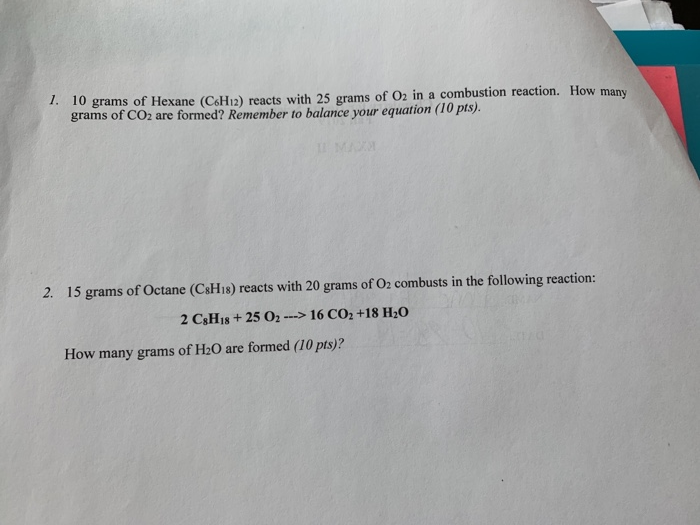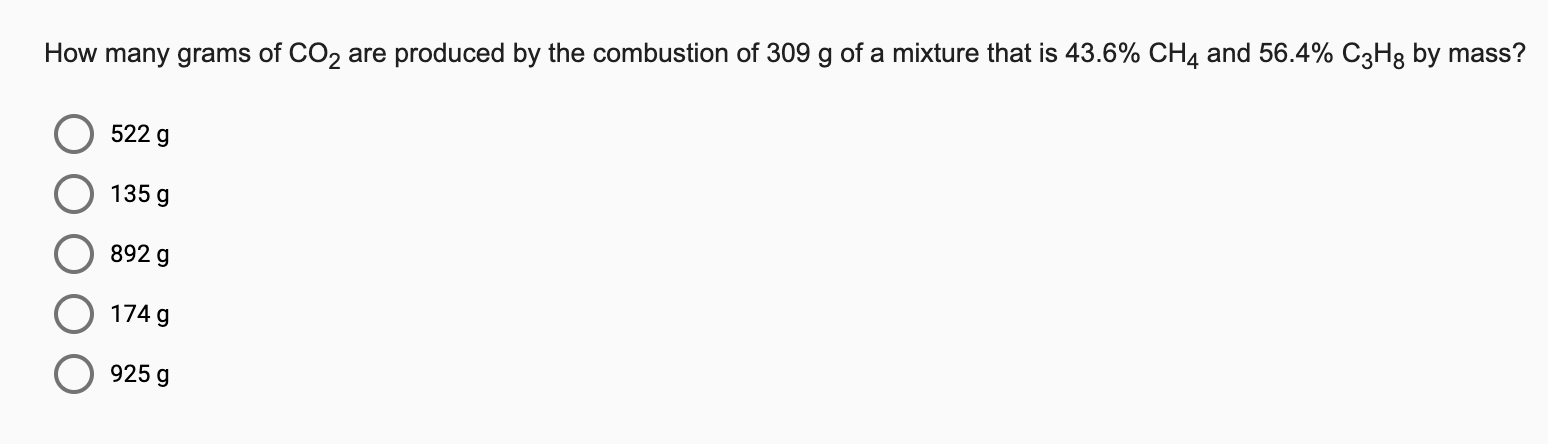Question

# If a mixture of 25.8 g C6H14 combusts, how many grams of O2 are needed to...

If a mixture of 25.8 g C6H14 combusts, how many grams of O2 are needed to complete the reaction with butane and how many grams of CO2 are produced?#### Earn Coins

Coins can be redeemed for fabulous gifts.

Similar Homework Help Questions
• ### When a mixture of 13.0 g of acetylene (C2H2) and 13.0 g of oxygen (O2) is...When a mixture of 13.0 g of acetylene (C2H2) and 13.0 g of oxygen (O2) is ignited, the resultant combustion reaction produces CO2 and H20. The unbalanced equation for this reaction is shown below: C2H2 + O2 +CO2 + H2O Part C How many grams of C2H, are present after the reaction is complete? VALO ? m = Бn Submit Request Answer

• ### to react 3 moles of glucose, how many moles of o2 are needed? when 540 g...

to react 3 moles of glucose, how many moles of o2 are needed? when 540 g of glucose are reacted, how many grams of co2 are produced?

• ### How many grams of CO2 are produced by the combustion of 344 g of a mixture...

How many grams of CO2 are produced by the combustion of 344 g of a mixture that is 33.6% CH4 and 66.4% C3H8 by mass?

• ### 1. 10 grams of Hexane (C6H12) reacts with 25 grams of O2 in a combustion reaction....1. 10 grams of Hexane (C6H12) reacts with 25 grams of O2 in a combustion reaction. How many grams of CO2 are formed? Remember to balance your equation (iopis). 2. 15 grams of Octane (C8H18) reacts with 20 grams of O2 combusts in the following reaction: 2 CxHj8 + 25 02 ---> 16 CO2 +18 H20 How many grams of H20 are formed (10 pts)?

• ### How many grams of CO2 are produced by the combustion of 309 g of a mixture...How many grams of CO2 are produced by the combustion of 309 g of a mixture that is 43.6% CH4 and 56.4% C3H7 by mass? 5229 O 1356 08929

• ### 1. [12.7 g C4H10] Butane, C4H10, is a common fuel. How many grams of butane can...

1. [12.7 g C4H10] Butane, C4H10, is a common fuel. How many grams of butane can be burned by 45.4 grams of oxygen?             2 C4H10 + 13 O2 ---> 8 CO2 + 10 H2O 2. [350. g NH4NO3] The fertilizer ammonium nitrate (NH4NO3) can be made by direct combination of ammonia with nitric acid:             NH3 + HNO3 ---->  NH4NO3 If 74.4 grams of ammonia (NH3) is reacted with nitric acid, how many grams of ammonium nitrate can be produced?...

• ### For hydrogen combustion, how many grams of O2 (g) are needed to react with 10.0 g...

For hydrogen combustion, how many grams of O2 (g) are needed to react with 10.0 g of H2 (g)?             2 H2 (g)      +        O2 (g)                                    2 H2O (l) 5.00 g O2 79.4 g O2 158.7 g O2 317.5 g O2

• ### 2C2H2+5O2=4CO2+2H2O Starting with 130g of C2H2, how many grams of O2 are required? How many grams...

2C2H2+5O2=4CO2+2H2O Starting with 130g of C2H2, how many grams of O2 are required? How many grams of CO2 are produced? How many grams of H2O are produced? If one produced 360g of CO2, how many grams of C2H2 did one start with? How many grams of O2 are required? How many grams of water were produced? Please show work!

• ### How many grams of O2(g) are needed to completely burn 35.9 g of C3H8(g)? C3H8 +...

How many grams of O2(g) are needed to completely burn 35.9 g of C3H8(g)? C3H8 + 5O2 ---> 3CO2 + 4H2O

• ### A mixture containing KClO3, K2CO3, KHCO3, and KCl was heated, producing CO2,O2, and H2O gases according...

A mixture containing KClO3, K2CO3, KHCO3, and KCl was heated, producing CO2,O2, and H2O gases according to the following equations: 2KClO3(s)2KHCO3(s)K2CO3(s)→→→2KCl(s)+3O2(g)K2O(s)+H2O(g)+2CO2(g)K2O(s)+CO2(g) The KCl does not react under the conditions of the reaction. 100.0 g of the mixture produces 1.70 g of H2O, 12.66 g of CO2, and 4.00 g of O2. (Assume complete decomposition of the mixture.) You may want to reference (Page) Section 3.7 while completing this problem. a. How many grams of KClO3 were in the original mixture?...